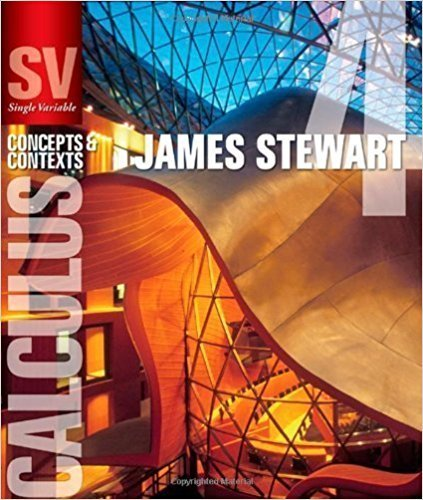×
×

# Solutions for Chapter 8.2: SERIES## Full solutions for Single Variable Calculus: Concepts and Contexts (Stewart's Calculus Series) | 4th Edition

ISBN: 9780495559726Solutions for Chapter 8.2: SERIES

Solutions for Chapter 8.2
4 5 0 351 Reviews
14
1
##### ISBN: 9780495559726

Single Variable Calculus: Concepts and Contexts (Stewart's Calculus Series) was written by and is associated to the ISBN: 9780495559726. This textbook survival guide was created for the textbook: Single Variable Calculus: Concepts and Contexts (Stewart's Calculus Series), edition: 4. This expansive textbook survival guide covers the following chapters and their solutions. Since 68 problems in chapter 8.2: SERIES have been answered, more than 20130 students have viewed full step-by-step solutions from this chapter. Chapter 8.2: SERIES includes 68 full step-by-step solutions.

Key Calculus Terms and definitions covered in this textbook
• Algebraic model

An equation that relates variable quantities associated with phenomena being studied

• Conic section (or conic)

A curve obtained by intersecting a double-napped right circular cone with a plane

• Conjugate axis of a hyperbola

The line segment of length 2b that is perpendicular to the focal axis and has the center of the hyperbola as its midpoint

• Constant of variation

See Power function.

• Cycloid

The graph of the parametric equations

• Difference of functions

(ƒ - g)(x) = ƒ(x) - g(x)

• Double-blind experiment

A blind experiment in which the researcher gathering data from the subjects is not told which subjects have received which treatment

• Exponential growth function

Growth modeled by ƒ(x) = a ? b a > 0, b > 1 .

• Higher-degree polynomial function

A polynomial function whose degree is ? 3

• Horizontal Line Test

A test for determining whether the inverse of a relation is a function.

• Imaginary part of a complex number

See Complex number.

• Implicitly defined function

A function that is a subset of a relation defined by an equation in x and y.

• Lower bound for real zeros

A number c is a lower bound for the set of real zeros of ƒ if ƒ(x) Z 0 whenever x < c

• Magnitude of an arrow

The magnitude of PQ is the distance between P and Q

• Parametric equations

Equations of the form x = ƒ(t) and y = g(t) for all t in an interval I. The variable t is the parameter and I is the parameter interval.

• Positive linear correlation

See Linear correlation.

• Product of functions

(ƒg)(x) = ƒ(x)g(x)

• Row echelon form

A matrix in which rows consisting of all 0’s occur only at the bottom of the matrix, the first nonzero entry in any row with nonzero entries is 1, and the leading 1’s move to the right as we move down the rows.

• Slope-intercept form (of a line)

y = mx + b

• Variation

See Power function.

×